# Methods and formulas for coefficients in Analyze Mixture Design

Select the method or formula of your choice.

## Coefficient (Coef)

Minitab uses least squares estimation to calculate the coefficients.

In matrix terms, the least squares estimates of the coefficients are:

b = (X'X)-1X'y

### Notation

TermDescription
Xdesign matrix
yresponse column
1. J.A. Cornell (1990). Experiments With Mixtures: Designs, Models, and the Analysis of Mixture Data, John Wiley & Sons.

## Standard error of the coefficient (SE Coef)

For simple linear regression, the standard error of the coefficient is:

The standard errors of the coefficients for multiple regression are the square roots of the diagonal elements of this matrix:

### Notation

TermDescription
xiith predictor value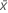mean of the predictor
Xdesign matrix
X'transpose of the design matrix
s2mean square error

## T-value

### Notation

TermDescription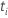test statistic for thecoefficient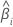estimated coefficient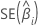standard error of theestimated coefficient

## P-value – Coefficients table

The two-sided p-value for the null hypothesis that a regression coefficient equals 0 is:

The degrees of freedom are the degrees of freedom for error, as follows:

np

### Notation

TermDescription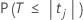The cumulative distribution function of the t distribution with degrees of freedom equal to the degrees of freedom for error.
tjThe t statistic for the jth coefficient.
nThe number of observations in the data set.
pThe sum of the degrees of freedom for the terms.

## Variance inflation factor (VIF)

The VIF can be obtained by regressing each predictor on the remaining predictors and noting the R2value.

### Formula

For predictor xj, the VIF is:

### Notation

TermDescription
R2( xj)coefficient of determination with xj as the response variable and the other terms in the model as the predictors
By using this site you agree to the use of cookies for analytics and personalized content.  Read our policy